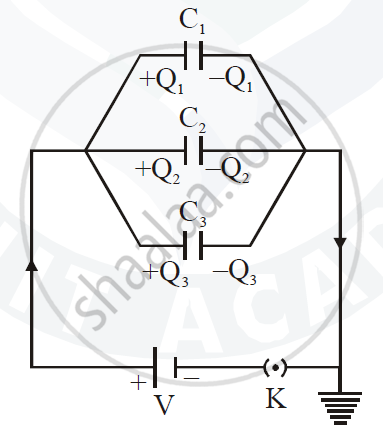Share

# Deduce an Expression for Equivalent Capacitance C When Three Capacitors C1, C2 and C3 Connected in Parallel. - ISC (Arts) Class 12 - Physics (Theory)

ConceptCombination of Capacitors

#### Question

Deduce an expression for equivalent capacitance C when three capacitors C1, C2 and C3 connected in parallel.

#### Solution

An expression for effective capacitance in the parallel grouping of capacitors :

Consider three capacitors of capacitance C1 , C2 and C3 are connected in parallel.

Let Q1, Q2 and Q3 be the charges deposited on the capacitors as shown in the figure.Suppose a potential difference ‘V ’ is applied across the combination. Then, the potential difference between the plates of each capacitor is V but charges on each capacitor are different. Since different current flows through different branches, so the charges are given by

Q_1 = C_1V.Q_2 = C_2V. Q_3 = C_3V    ....(i)

From the principle of conservation of charge

Q = Q_1 + Q_2 + Q_3

Q = C_1V + C_2V + C_3V      [From equation (i)]

∴ Q = V(C_1 + C_2 + C_3)

If these capacitors are replaced by a single capacitor of capacity CP such that Q = C_PV  then using equation (ii) we have,

C_PV = V(C_1+C_2+C_3)

C_P = C_1+C_2+C_3

Is there an error in this question or solution?

#### Video TutorialsVIEW ALL 

Solution Deduce an Expression for Equivalent Capacitance C When Three Capacitors C1, C2 and C3 Connected in Parallel. Concept: Combination of Capacitors.
S